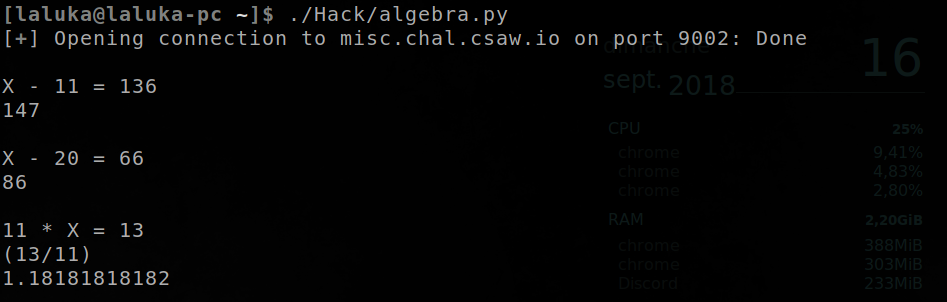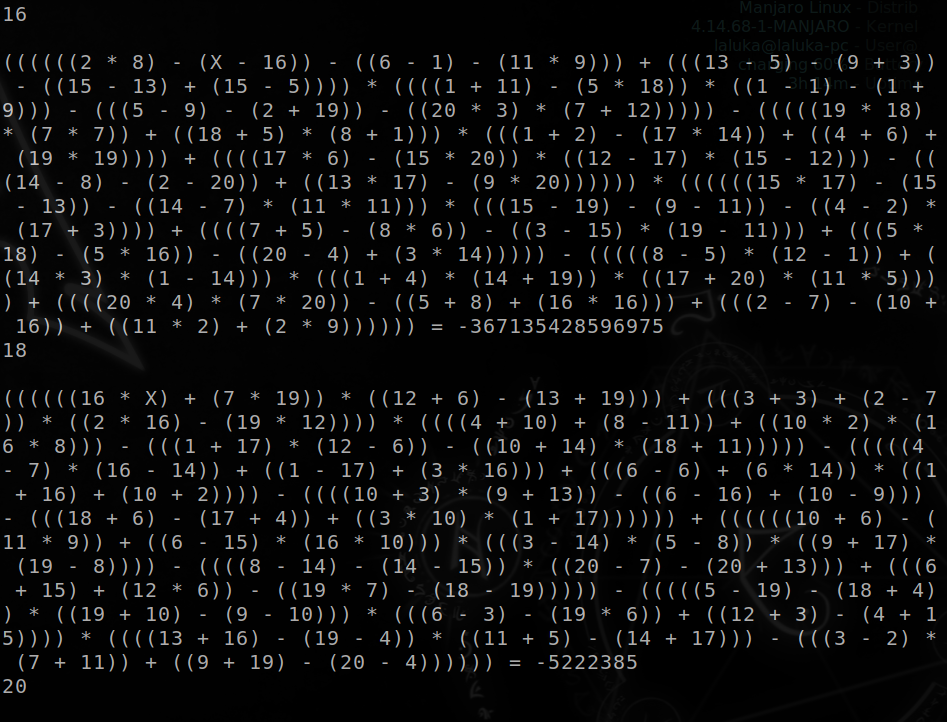# Algebra

CSAW'18 CTF Qualification - MISC (100 pts).

# CSAW’18 CTF Qualification: Algebra

Event Challenge Category Points Solves
CSAW’18 CTF Qualification Algebra MISC 100 410

### Description

Are you a real math wiz?

nc misc.chal.csaw.io 9002

### TL;DR

This challenge consists in equations being thrown at us that we have to solve and send the result in order to get the flag. Python and sage, guide us please !

### Methology

A friend I was talking with while solving this chall told me :

Well, the equations are simple, let’s write a quick script to solve it !

Hearing that, I went like :

Hum… Hum no. That’s not how it works, they wouldn’t give 100 points for that…

In fact, during CSAW 2017 quals, there was an other Misc I worked on, and the questions (bank card number to compute) were getting harder and harder, so I knew we had to find a better way to solve it automatically. So I took my worst coding skills, and used `popen` and `eval` like I it was my last day on earth. Here’s the result, 10mn coding ! :)

``````#!/usr/bin/env python2

from pwn import *
import os

r = remote("misc.chal.csaw.io", 9002)

while True:
try:
data = r.recvuntil("equal?: ")
line = [line for line in data.splitlines() if "=" in line]
print "\n" + line
out = os.popen("sage -c \"var('X'); print solve([" + line.replace("=", "==") + "], X)\"").read()
answer = out.split("== ").split("\n")
print answer
if "/" in answer: # Sage does not simplify, so python will do it ! :)
answer = eval(answer.replace("/", "/float(") + ")")
print answer
r.sendline(str(answer))
except:
print "out", out # In case of failure, please tell us why...
r.interactive()
``````

Just so you can laugh a bit, here are the first and last equations…

If I had to code something that can handle that… I would… I wouldn’t.The flag is : flag{y0u_s0_60od_aT_tH3_qU1cK_M4tH5}

-Laluka